# What is Field Effect Transistor (FET) | Types

Want create site? Find Free WordPress Themes and plugins.

Bipolar junction transistor (BJT) is a current-driven device. The input current to the device controls the output current. From the point of view of efficiency this type of transistor consumes a relatively high amount of energy due to high current, which converts to heat; thus, the efficiency is low. There are other types of a transistor with better energy efficiency. This is due to their higher inherent resistance. A field effect transistor (FET) is another type of transistor that, because of its advantages over the junction transistor, is widely used in industrial and domestic electronic appliances.

Transistor circuit resistances for a field effect transistor are much larger than their counterparts in BJTs. The current, consequently, is much lower, which leads to much smaller power consumption in a circuit employing this category of the transistor.

Field effect transistor (FET): Type of transistor, still made of semiconductor materials, based on a different structure and a different mechanism of operation than the junction transistor. It has a channel or passage around which an electric field can be developed and through which electrons flow. The flow of electrons can be controlled by adjusting the polarity and intensity of the field.

A basic difference between a FET and a junction transistor is that a FET has only one P-N junction. The structure and the manufacturing process of a FET are different from those of a bipolar junction transistor.

In a field effect transistor, the main body can be N-type or P-type material in which a channel from the opposite type material is diffused. If the channel material is made of N material, then the product is called N-channel FET, and if the channel is made of P-type semiconductor, the FET is called P-channel FET.

A FET is a voltage-driven transistor, dissimilar to BJT, which is a current-driven device. The reason it is called field effect transistor is that the current through the channel is affected by an electrostatic field formed around the channel. The strength of this field can control the flow of the electric carriers in the channel.

A schematic of the active area of a FET and its three terminals are shown in Figure 1. In practice, a transistor is very small, and all its elements are made on a body that itself is a semiconductor and is part of the transistor elements.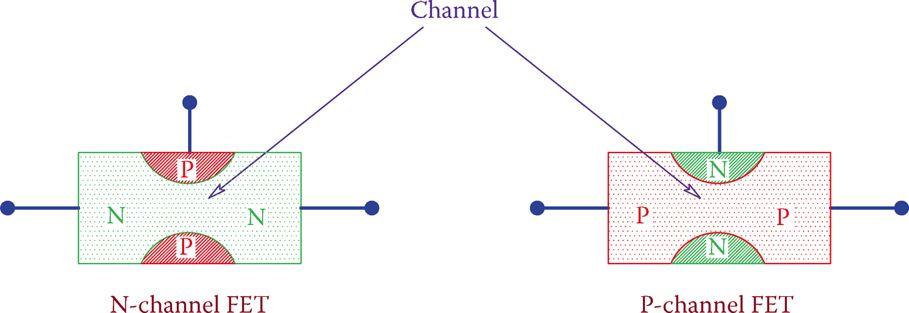Figure 1 Schematic of FET active parts.

The three elements of a field effect transistor have names different than those for BJT. When a FET conducts, electrons move through the channel. One side of the channel is called the source (counterpart of emitter) and the other side is called the drain (counterpart to collector).

The channel is physically surrounded by the opposite type semiconductor material, which can influence the extent (effective size) of the channel, thus introducing less or more resistance for the flow of electrons. This control action is carried out by the gate.

The channel size (effect size not the physical size) is governed by the voltage applied to the gate and not the current through the gate. This is why a FET is a voltage-driven device.

Source: (in semiconductors) One of the three terminals in a field effect transistor, comparable with the emitter in a junction transistor.

Drain: One of the three terminals in a field effect transistor (FET) counterpart of the collector in a junction transistor.

Gate: Special connection in some semiconductor devices that upon receiving an appropriate signal (in the form of a voltage or pulse) allows controlling of the device, including turning it on and off.

There are many types of FETs and in some (with a symmetrical geometry for the channel) the designation of source and drain is governed by biasing.

In some others, the channel does not have a symmetrical shape (structured different in the process of manufacturing) and one side of the channel is designated as the source and the other side is the drain. Figure 2 illustrates the schematic of the structure of a FET.

In comparison with a bipolar junction transistor the source, drain, and gate are the counterparts for the emitter, collector, and base, respectively. Also, the N-channel FET is the counterpart of the NPN transistor and the P-channel FET corresponds to a PNP transistor.

Physically, FETs are made similar to BJTs, and there is no way to distinguish one from the other just by looking. One must pay attention to the name tag and the manufacturer’s data sheets.

Apart from a number of differences between these two categories of transistors, all that can be done by bipolar transistors, such as amplification, can also be done by FETs.

In bipolar transistors, we have a common emitter, common base, and a common collector amplifier. Likewise, in FETs, we have common-source, common-gate, and common-drain amplifiers, respectively.

FETs can be used for amplification. Analogous to BJTs, it is possible to have common-source, common-drain, and common-gate amplifiers.

In the same way that an NPN transistor is equally applicable to a PNP transistor, whatever applies to an N-channel FET is true for a P-channel FET, too. The polarity of biasing and operating voltages, accordingly, are the reverse of each other for the N-channel and P-channel FETs. In what follows, we consider an N-channel field effect transistor.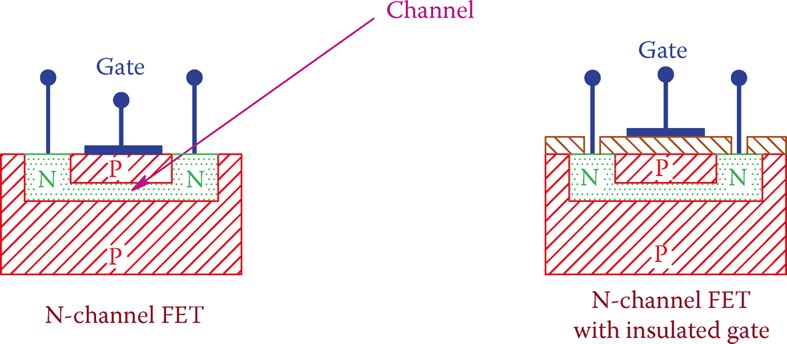Figure 2 Schematic structure of FET.

## Field Effect Transistor (FET) Types

Field effect transistors are initially categorized into two types, junction field effect transistor, and insulated gate field effect transistor.

The first category is referred to by its abbreviation JFET. The second category is known as MOSFET because of the material used for its gate insulation being a metal-oxide semiconductor. Thus, a more common name for the second category is the metal-oxide-semiconductor field effect transistor.

Junction field effect transistor: One type of field effect transistor (FET) in which the gate is not insulated. Those with insulated gates are more advanced and more common.

Insulated gate field effect transistor: Type of FET with insulated gate, like MOSFET.

Metal-oxide-semiconductor: Type of semiconductor device in which metal oxide is used for insulation layers.

Metal-oxide-semiconductor field effect transistor (MOSFET): Type of field effect transistor in which metal oxide (like SiO2) is employed for insulation of the gate.

The two types are different in structure and there is a difference between the ways they can function. In JFET the isolation between the gate and channel is a reverse biased PN junction. In MOSFET a layer of metal oxide is used for this purpose. This is schematically depicted in Figure 2.

In addition to the final categorization of each type to N-channel and P-channel, MOSFETs have subcategories, as discussed later. Figure 3 indicates the possible categories of field effect transistors.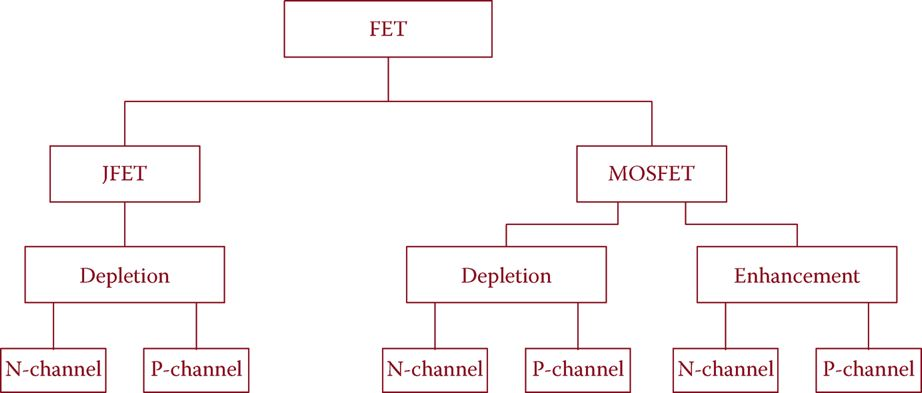Figure 3 Tree of various families of field effect transistors.

As can be seen, the JFET family is associated with depletion, whereas MOSFET family is associated with depletion and enhancement. These (depletion and enhancement) are the modes of operation for each family; that is, the mechanism under which each category internally functions. They are described below.

In the depletion region around a P-N joint the electrons that have moved to this region reject and prevent other electrons to enter or pass through the depletion layer. This is what can happen in the channel of a FET.

Suppose that the two sides of a channel formed by an N-type semiconductor are connected to a voltage, as shown in Figure 4. Electrons are attracted to the + side (from left to right).

The channel material is a semiconductor, and it exhibits some resistance, behaving like a resistor. Consider three cases:

(1) there is no voltage on the gate,

(2) the gate is connected to a negative voltage, and

(3) the gate is connected to a positive voltage.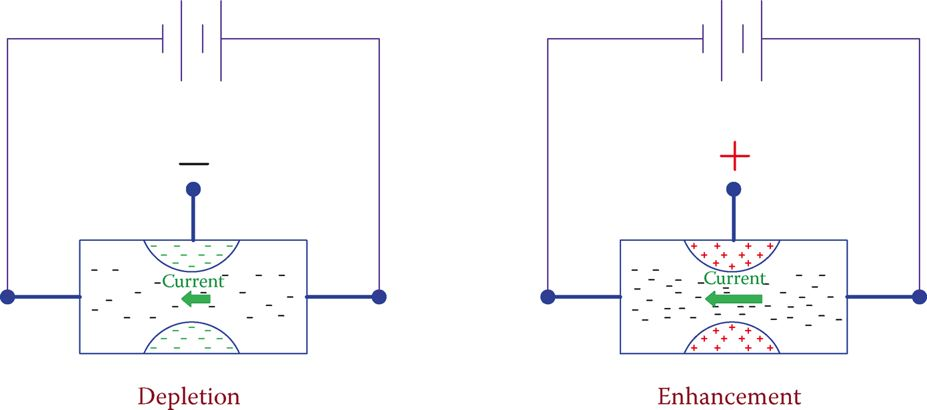Figure 4 Depletion and enhancement in field effect transistor.

Case 1

When there is no voltage on the gate, a current can flow in the channel due to the voltage difference between the two sides of the channel and based on the resistance of the channel.

Case 2

If now a negative voltage is connected to the gate, a negative field will be formed around the channel, as shown in Figure 4. The effect of this field is to reject electrons and decrease the current. This is the case called depletion because the negative field depletes the electrons in the channel. The stronger the field is, the lower the number of electrons passing.

Case 3

When the gate is connected to a positive voltage, a positive field is formed around the channel. The effect on the electrons is to attract them and, as a result, help them move through the channel. This is called enhancement; the motion of electrons is enhanced.

In both cases (depletion and enhancement) the stronger the field is the larger is the effect on the electron flow. As depicted in Figure 3, JFETs work only based on depletion, but MOSFETs can work either in the depletion mode or the enhancement mode.

Enhancement: One of the two methods to control the flow of electrons in the channel of a field effect transistor (FET).

In a FET, by changing the voltage on the gate the current between the source and drain can be controlled.

## Junction Field Effect Transistor (JFET)

Although JFETs and MOSFETs are both field effect transistors, they have different symbols in the circuit schematics. The symbols for JFET and a comparison with their counterparts in bipolar junction transistors are shown in Figure 5.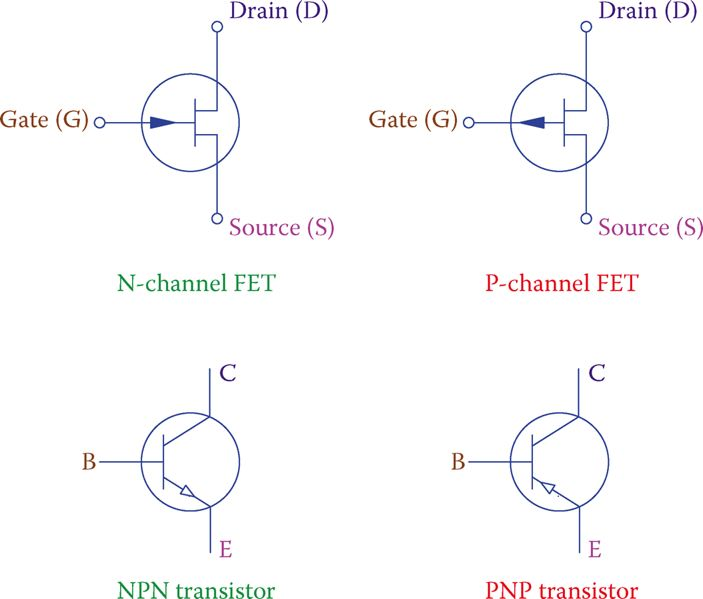Figure 5 Symbols for N-channel and P-channel field effect transistors.

## N-Channel JFET Characteristics

For an N-channel JFET, the drain voltage must be more positive than the source voltage; that is, the drain must be at a higher voltage with respect to the source. This is similar to the polarity for an NPN bipolar junction transistor where the collector-emitter junction must be forward biased.

The gate for this type FET, nevertheless, must be negative with respect to the source; that is, the gate-source junction (P-type material in the gate and N-type for the channel) must have a reverse bias.

The gate must have a lower voltage than the source. For this type of FET at the most, the gate can be shorted to the source and have a voltage equal to that of the source. Gate voltage going more positive than the source voltage is harmful to the JFET and can damage it.

For a P-channel JFET, the reverse is true. Typically acceptable voltages are shown in Figure 6.

For N-channel JFET the gate must be negative with respect to the source.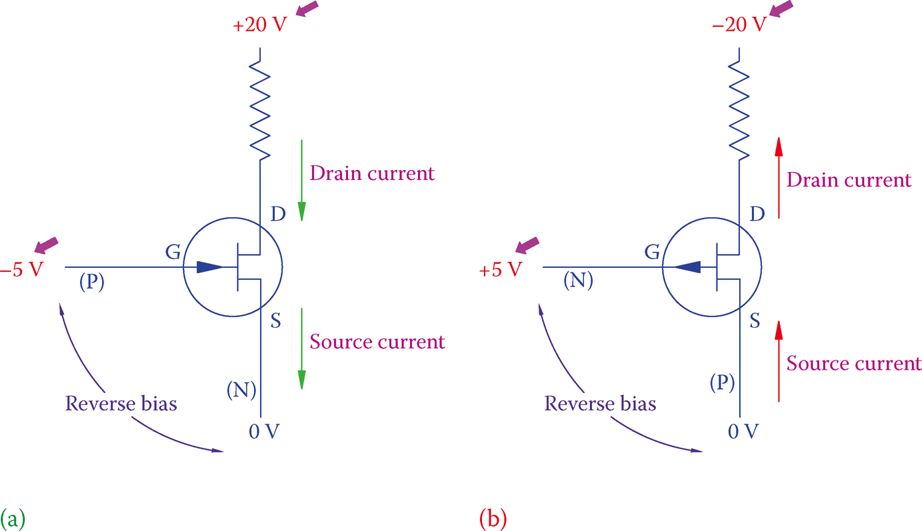Figure 6 Voltage and bias relationships for JFET: (a) N channel and (b) P channel.

In an N-channel JFET when a negative voltage is applied to the gate the effect is to introduce more resistance to the source-drain electric flow, as if the channel becomes narrower. This is due to the growth of the depletion region where the N-type and P-type materials meet.

If the gate negative voltage is sufficiently high, then the channel entirely blocks and the current drops to zero. (The transistor goes to the cutoff state.) In this respect, the highest source current corresponds to the case when the gate and the source have the same voltage.

Note that the gate-source junction is reverse biased. Consequently, there is no current flowing between the source and the gate. Unlike the case of a BJT, therefore, the source current and the drain current are the same.

The voltage at the gate is only to maintain the electrostatic field around the channel. Similar to a BJT, various voltages and currents in a FET are denoted by their corresponding element. These are shown in Figure 7. These definitions are good for MOSFET, too.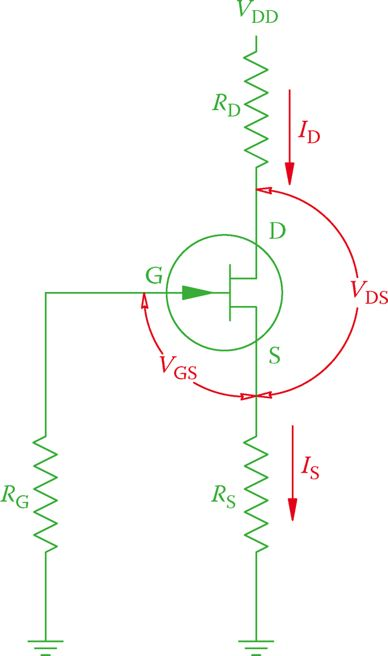Figure 7 Definition of FET circuit components.

The required bias condition exemplified in Figure 6 necessitates that both positive and negative voltages be available from a power supply. Having two power supplies is undesirable, and normally one power supply is used to power a transistor.

In the same way, as it was for BJTs, appropriate biasing for FETs can be obtained by fix bias, self-bias or voltage divider bias and are shown in Figure 8.

In a field effect transistor, the source current and the drain current are equal.

Note that in the voltage divider biasing method the voltage at G is determined by the values of RG and $R_{G}^{‘}$. This value is positive for a positive VDD. RG

The voltage at S, however, is determined by the values of RS and IS. Correct gate-source bias can be obtained by choosing RS appropriately.

The incorrect magnitude of RS can make the voltage at S smaller than the gate voltage (in an N-channel transistor), which can cut off the FET; so, it is important that RS is correctly selected. When dealing with AC signals that cause IS to vary between a minimum and a maximum value, the transistor can go to the cutoff for some values of IS.Figure 8 Biasing methods for FET: (a) fixed bias, (b) self-bias, and (c) voltage divider bias.

## Metal Oxide Semiconductor Field Effect Transistor (MOSFET)

The metal oxide semiconductor field effect transistor (MOSFET) is a FET whose gate is insulated from the main body of the transistor by a layer of metal oxide semiconductor (such as SiO2). This layer is very thin, and for this reason, MOSFETs are prone to get damaged easily if they are subject to voltages higher than their ratings.

They are also very sensitive to static electricity, and they must be handled with care and sufficient protection from receiving static electricity in order not to get damaged.

In addition to the gate being insulated, one more difference between JFET and MOSFET is the channel. While in a JFET, the channel is made of the same material as the source and drain, a MOSFET has no channel or has a smaller channel made of a semiconductor material that is much less doped than those of the source and drain.

As pointed out earlier, there are two types of MOSFET based on the mechanism that they function, depletion and enhancement.

The first type is called D-MOSFET. It has a channel, and it can work both in depletion and enhancement mode (for this reason sometimes it is called DE-MOSFET).

The second type, called E-MOSFET, can only work in the enhancement mode. Figure 10 schematically depicts the structural difference between the two types.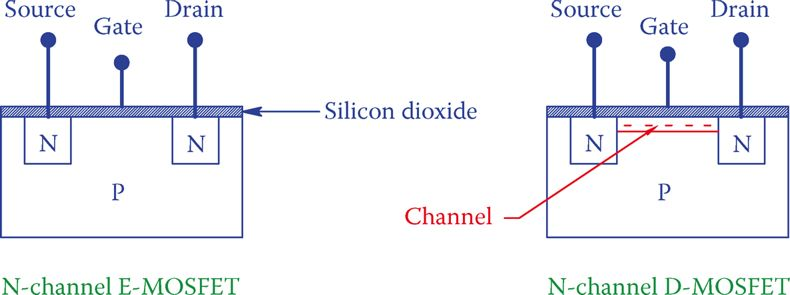Figure 10 Structural difference between D-MOSFET and E-MOSFET.

An E-MOSFET is an off device, meaning that when no voltage is applied to the gate (VGS = 0), the transistor is in the cutoff mode. If then a positive voltage is applied to the gate, it develops a current between the source and the drain. This current can be controlled by changing the gate voltage.

In the D-MOSFET, however, there is a current IDS between the drain and source even when VGS = 0 (when a VDS exists between the drain and the source). Then IDS can be lowered (working in the depletion mode) by having a negative voltage applied to the gate or it can be increased (working in the enhancement mode) by applying a positive voltage to the gate.

Symbols for the main categories of MOSFETs are shown in Figure 11. There are more varieties of MOSFET, but we do not consider them here.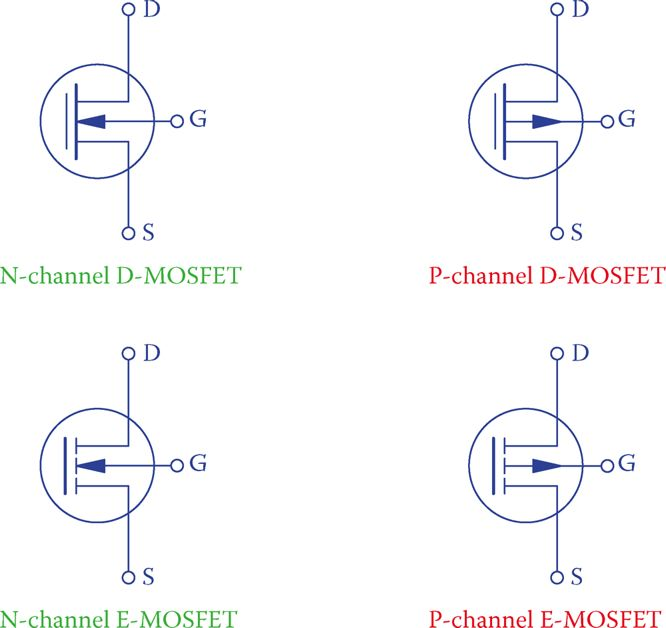Figure 11 MOSFET symbols.

A MOSFET has a high input impedance. This is a definite advantage over the BJT and JFET because it implies low input current and very low power dissipation.

Also, it is more appropriate for connecting to devices with a high output impedance. Other advantages that have made the use of MOSFETs more popular than BJTs are (1) insensitivity to temperature change and (2) high-frequency capability (fast switching).

Did you find apk for android? You can find new Free Android Games and apps.